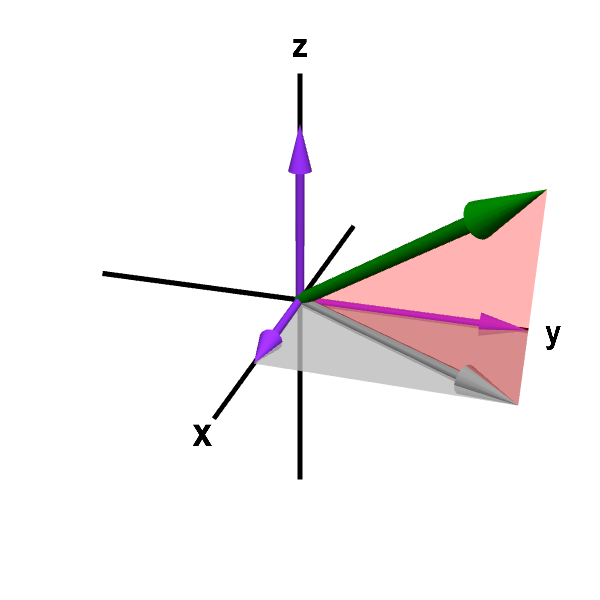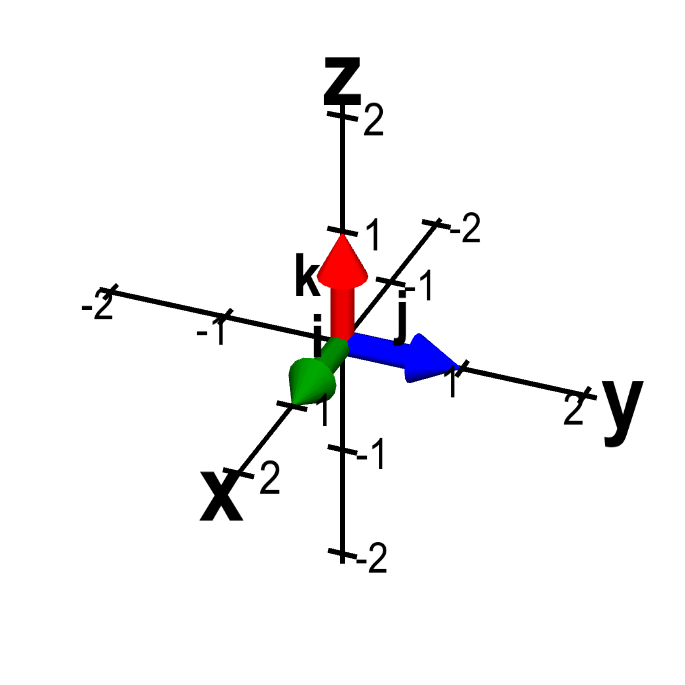# Math Insight

### Vectors in two- and three-dimensional Cartesian coordinates

In the introduction to vectors, we discussed vectors without reference to any coordinate system. By working with just the geometric definition of the magnitude and direction of vectors, we were able to define operations such as addition, subtraction, and multiplication by scalars. We also discussed the properties of these operation.

Often a coordinate system is helpful because it can be easier to manipulate the coordinates of a vector rather than manipulating its magnitude and direction directly. When we express a vector in a coordinate system, we identify a vector with a list of numbers, called coordinates or components, that specify the geometry of the vector in terms of the coordinate system. Here we will discuss the standard Cartesian coordinate systems in the plane and in three-dimensional space.

#### Vectors in the plane

We assume that you are familiar with the standard $(x,y)$ Cartesian coordinate system in the plane. Each point $\vc{p}$ in the plane is identified with its $x$ and $y$ components: $\vc{p} = (p_1,p_2)$.

To determine the coordinates of a vector $\vc{a}$ in the plane, the first step is to translate the vector so that its tail is at the origin of the coordinate system. Then, the head of the vector will be at some point $(a_1,a_2)$ in the plane. We call $(a_1,a_2)$ the coordinates or the components of the vector $\vc{a}$. We often write $\vc{a} \in \R^2$ to denote that it can be described by two real coordinates.

Using the Pythagorean Theorem, we can obtain an expression for the magnitude of a vector in terms of its components. Given a vector $\vc{a}=(a_1,a_2)$, the vector is the hypotenuse of a right triangle whose legs are length $a_1$ and $a_2$. Hence, the length of the vector $\vc{a}$ is $$\|\vc{a}\| = \sqrt{a_1^2+a_2^2}.$$

As an example, consider the vector $\vc{a}$ represented by the line segment which goes from the point $(1,2)$ to the point $(4,6)$. Can you calculate the coordinates and the length of this vector?

To find the coordinates, translate the line segment one unit left and two units down. The line segment begins at the origin and ends at $(4-1,6-2) = (3,4)$. Therefore, $\vc{a} = (3,4)$. The length of $\vc{a}$ is $\|\vc{a}\| = \sqrt{3^2+4^2} = 5$.

The below applet, repeated from the vector introduction, allows you to explore the relationship between a vector's components and its magnitude.

The magnitude and direction of a vector. The blue arrow represents a vector $\vc{a}$. The two defining properties of a vector, magnitude and direction, are illustrated by a red bar and a green arrow, respectively. The length of the red bar is the magnitude $\|\vc{a}\|$ of the vector $\vc{a}$. The green arrow always has length one, but its direction is the direction of the vector $\vc{a}$. The one exception is when $\vc{a}$ is the zero vector (the only vector with zero magnitude), for which the direction is not defined. You can change either end of $\vc{a}$ by dragging it with your mouse. You can also move $\vc{a}$ by dragging the middle of the vector; however, changing the position of the $\vc{a}$ in this way does not change the vector, as its magnitude and direction remain unchanged.

The vector operations we defined in the vector introduction are easy to express in terms of these coordinates. If $\vc{a}=(a_1,a_2)$ and $\vc{b}=(b_1,b_2)$, their sum is simply $\vc{a}+\vc{b}=(a_1+b_1,a_2+b_2)$, as illustrated in the below figure. It is also easy to see that $\vc{b}-\vc{a} = (b_1-a_1,b_2-a_2)$ and $\lambda \vc{a} = (\lambda a_1, \lambda a_2)$ for any scalar $\lambda$.

The below applet, also repeated from the vector introduction, allows you to explore the relationship between the geometric definition of vector addition and the summation of vector components.

The sum of two vectors. The sum $\vc{a}+\vc{b}$ of the vector $\vc{a}$ (blue arrow) and the vector $\vc{b}$ (red arrow) is shown by the green arrow. As vectors are independent of their starting position, both blue arrows represent the same vector $\vc{a}$ and both red arrows represent the same vector $\vc{b}$. The sum $\vc{a}+\vc{b}$ can be formed by placing the tail of the vector $\vc{b}$ at the head of the vector $\vc{a}$. Equivalently, it can be formed by placing the tail of the vector $\vc{a}$ at the head of the vector $\vc{b}$. Both constructions together form a parallelogram, with the sum $\vc{a}+\vc{b}$ being a diagonal. (For this reason, the commutative law $\vc{a}+\vc{b}=\vc{b}+\vc{a}$ is sometimes called the parallelogram law.) You can change $\vc{a}$ and $\vc{b}$ by dragging the yellow points.

You may have noticed that we use the same notation to denote a point and to denote a vector. We don't tend to emphasize any distinction between a point and a vector. You can think of a point as being represented by a vector whose tail is fixed at the origin. You'll have to figure out by context whether or not we are thinking of a vector as having its tail fixed at the origin.

Another way to denote vectors is in terms of the standard unit vectors denoted $\vc{i}$ and $\vc{j}$. A unit vector is a vector whose length is one. The vector $\vc{i}$ is the unit vector in the direction of the positive $x$-axis. In coordinates, we can write $\vc{i}=(1,0)$. Similarly, the vector $\vc{j}$ is the unit vector in the direction of the positive $y$-axis: $\vc{j}=(0,1)$. We can write any two-dimensional vector in terms of these unit vectors as $\vc{a}=(a_1,a_2) = a_1\vc{i}+a_2\vc{j}$.

#### Vectors in three-dimensional space

In three-dimensional space, there is a standard Cartesian coordinate system $(x,y,z)$. Starting with a point which we call the origin, construct three mutually perpendicular axes, which we call the $x$-axis, the $y$-axis, and the $z$-axis. Here is one way to picture these axes. Stand near the corner of a room and look down at the point where the walls meet the floor. Then, the floor and the wall to your left intersect in a line which is the positive $x$-axis. The floor and the wall to your right intersect in a line which is the positive $y$-axis. The walls intersect in a vertical line which is the positive $z$-axis. These positive axes are depicted in the below applet labeled by $x$, $y$, and $z$. The negative part of each axis is on the opposite side of the origin, where the axes intersect.Three-dimensional Cartesian coordinate axes. A representation of the three axes of the three-dimensional Cartesian coordinate system. The positive $x$-axis, positive $y$-axis, and positive $z$-axis are the sides labeled by $x$, $y$ and $z$. The origin is the intersection of all the axes. The branch of each axis on the opposite side of the origin (the unlabeled side) is the negative part. You can drag the figure with the mouse to rotate it.

We have set the relative locations of the positive $x$, $y$, and $z$-axis to make the coordinate system a right-handed coordinate system. Note that if you curl the fingers of your right hand from the positive $x$-axis to the positive $y$-axis, the thumb of your right hand points in the direction of the positive $z$-axis.

If you switched the locations of the positive $x$-axis and positive $y$-axis, then you would end up having a left-handed coordinate system. If you do that, you will be living in a mathematical universe in which some formulas will differ by a minus sign from the formula in the universe we are using here. Your universe will be just as valid as ours, but there will be lots of confusion. We suggest you live in our universe while studying from these pages.

With these axes any point $\vc{p}$ in space can be assigned three coordinates $\vc{p}=(p_1,p_2,p_3)$. For example, given the above corner-of-room analogy, suppose you start at the corner of the room and move four meters along the $x$-axis, then turn left and walk three meters into the room. If you are two meters tall, then the top of your head is at the point $(4,3,2)$.

Just as in two-dimensions, we assign coordinates of a vector $\vc{a}$ by translating its tail to the origin and finding the coordinates of the point at its head. In this way, we can write the vector as $\vc{a}=(a_1,a_2,a_3)$. We often write $\vc{a} \in \R^3$ to denote that it can be described by three real coordinates. Sums, differences, and scalar multiples of three-dimensional vectors are all performed on each component. If $\vc{a}=(a_1,a_2,a_3)$ and $\vc{b}=(b_1,b_2,b_3)$, then $\vc{a}+\vc{b}=(a_1+b_1,a_2+b_2,a_3+b_3)$, $\vc{b}-\vc{a}=(b_1-a_1,b_2-a_2,b_3-a_3)$, and $\lambda\vc{a}=(\lambda a_1, \lambda a_2, \lambda a_3)$.A vector in three-dimensional space. A representation of a vector $\vc{a}=(a_1,a_2,a_3)$ in the three-dimensional Cartesian coordinate system. The vector $\vc{a}$ is drawn as a green arrow with tail fixed at the origin. You can drag the head of the green arrow with your mouse to change the vector. To help show the three dimensional perspective, a pink triangle connects the vector to its projection $(a_1,a_2,0)$ in the $xy$-plane (gray arrow). Purple vectors show the projections of $\vc{a}$ on each axis and represent the coordinates $a_1$, $a_2$, and $a_3$. You can also drag the heads of the purple vectors to change just one of the coordinates of the vector. Or drag the head of the gray vector in the $xy$-plane to change just the $x$ and $y$ coordinates.

Just as in two dimensions, we can also denote three-dimensional vectors is in terms of the standard unit vectors, $\vc{i}$, $\vc{j}$, and $\vc{k}$. These vectors are the unit vectors in the positive $x$, $y$, and $z$ direction, respectively. In terms of coordinates, we can write them as $\vc{i}=(1,0,0)$, $\vc{j}=(0,1,0)$, and $\vc{k}=(0,0,1)$. We can express any three-dimensional vector as a sum of scalar multiples of these unit vectors in the form $\vc{a}=(a_1,a_2,a_3) = a_1\vc{i}+a_2\vc{j}+a_3\vc{k}$.The standard unit vectors in three dimensions. The standard unit vectors in three dimensions, $\vc{i}$ (green), $\vc{j}$ (blue), and $\vc{k}$ (red) are length one vectors that point parallel to the $x$-axis, $y$-axis, and $z$-axis respectively. Moving them with the mouse doesn't change the vectors, as they always point toward the positive direction of their respective axis.

What is the length of the vector $\vc{a}=(a_1,a_2,a_3)$? We can decompose the vector into $(a_1,a_2,a_3) = (a_1,a_2,0)+(0,0,a_3)$, where the two vectors on the right hand side correspond to the two green line segments in the above applet. These two line segments form a right triangle whose hypotenuse is the vector $\vc{a}$ (the blue line segment). The first vector can be thought of as a two dimensional vector, so its length is $\|(a_1,a_2,0)\| = \|(a_1,a_2)\| = \sqrt{a_1^2+a_2^2}$. The second vector's length is $\|(0,0,a_3)\| = |a_3|$. Therefore, by the Pythagorean Theorem, the length of $\vc{a}$ is $$\|\vc{a}\| = \sqrt{\|(a_1,a_2,0)\|^2 + \|(0,0,a_3)\|^2} = \sqrt{a_1^2+a_2^2+a_3^2}.$$

#### Going beyond three dimensions?

We can easily visualize two or three dimensions by drawing pictures of a plane or of space. If we represent a vector by a list of numbers such as $(a_1,a_2) \in \R^2$ and $(a_1,a_2,a_3) \in \R^3$, we can easily go beyond three dimensions into four dimensions $(a_1,a_2,a_3,a_4) \in \R^4$ or even to arbitrary dimension such as $n$-dimensions $(a_1,a_2, \ldots, a_n) \in \R^n$, where $n$ is some positive integer.

Going to higher dimension is easy with lists of numbers, though of course high-dimensional vectors are not easy (not possible?) to visualize. You can read more about high-dimensional vectors or check out examples of $n$-dimensional vectors that illustrate how going to dimensions higher than three can be useful in many situations.

`